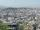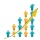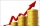# Population

What is the population of the city with 3% annual growth, if in 10 years the city will have 60,000 residents?

Correct result:

n =  44646

#### Solution:We would be pleased if you find an error in the word problem, spelling mistakes, or inaccuracies and send it to us. Thank you!Tips to related online calculators
Do you want to convert time units like minutes to seconds?

## Next similar math problems:

• The city 3The city has 22,000 residents. How long it is expected to have 25,000 residents if the average annual population growth is 1.4%?
• DemographicsThe population grew in the city in 10 years from 42000 to 54500. What is the average annual percentage increase of population?
• PopulationThe town has 65,000 inhabitants. 40 years ago there were 157,000. How many people will live in town in 10 years if the average rate in population is as in previous years?
• Compound interestCompound interest: Clara deposited CZK 100,000 in the bank with an annual interest rate of 1.5%. Both money and interest remain deposited in the bank. How many CZK will be in the bank after 3 years?
• The cityAt the end of 2010 the city had 248000 residents. The population increased by 2.5% each year. What is the population at the end of 2013?
• Population growthHow many people will be on Earth from two people for 5,000 years, if every couple has always 4 children, (2 boys and 2 girls) at the age of 25-35, and every man will live 75 years?
• BankPaul put 10000 in the bank for 6 years. Calculate how much you will have in the bank if he not pick earned interest or change deposit conditions. The annual interest rate is 3.5%, and the tax on interest is 10%.
• Growth of woodThe annual growth of wood in the forest is estimated at 2%. In how many years will make the forest volume double?
• The city 2Today lives 60000 citizens in city. How many citizens can we expect in 8 years if their annual increase is 2.9%?
• Common ratioIf 200 units of a commodity are consumed in a first year, and if the annual rate of increase in consumption is 5% (a) what amount is consumed in the 8th year; (b) in the first 15 years?
• If you 3If you deposit $4500 at 5% annual interest compound quarterly, how much money will be in the account after 10 years? • SavingsThe depositor regularly wants to invest the same amount of money in the financial institution at the beginning of the year and wants to save 10,000 euros at the end of the tenth year. What amount should he deposit if the annual interest rate for the annua • DepositIf you deposit 719 euros the beginning of each year, how much money we have at 1.3% (compound) interest after 9 years? • What isWhat is the annual percentage increase in the city when the population has tripled in 20 years? • Present valueA bank loans a family$90,000 at 4.5% annual interest rate to purchase a house. The family agrees to pay the loan off by making monthly payments over a 15 year period. How much should the monthly payment be in order to pay off the debt in 15 years?A radioactive material loses 10% of its mass each year. What proportion will be left there after n=6 years?My savings of php 90,000 in a bank earns 6% interest in a year. If i will deposit additional php 10,000 at the end of 6 months, how much money will be left if i withdraw php 25,000 after a year?